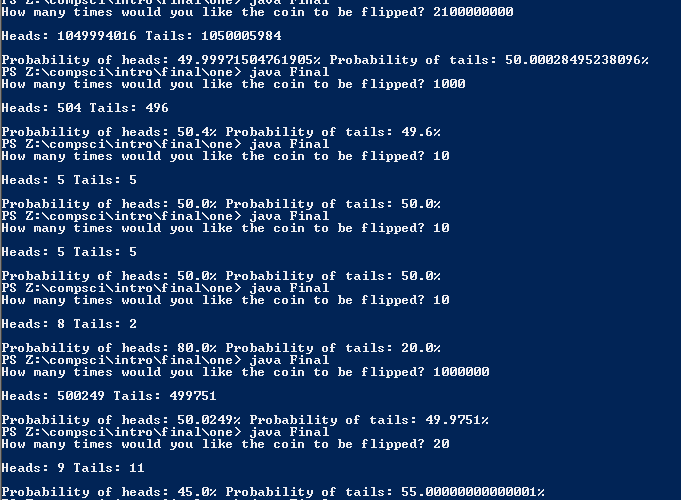# Semester One Final

## Code

```    ///Name: Tim Gibson
///Period: 6
///Project Name: Semester One Final
///File Name: Final.java
///Date Finished: 1/22/15

import java.util.Scanner;
import java.util.Random;

public class Final
{
public static void main ( String[] args )
{
Scanner kb = new Scanner(System.in);
Random r = new Random();
int ht, nof, t, h; //ht for heads or tales nof is number of flips, t for tails, h for heads
double poh, pot; //probabilty of heads/tails
t = 0;
h = 0;//have to be initialized
do
{

System.out.print("How many times would you like the coin to be flipped? ");
nof = kb.nextInt();

if ( nof <= 0 || nof > 2100000000 )
System.out.println("Improper number of flips. Try again.");
} while ( nof <= 0 || nof > 2100000000 );

for ( int x = 1; x <= nof; x++)
{
ht = 1 + r.nextInt(2);

if ( ht == 1 ) //heads
h++;
else if ( ht == 2 ) //tails
t++;
}

poh = ((double)h / nof) * 100;
pot = ((double)t / nof) * 100;
System.out.println( "\nHeads: " + h + " Tails: " + t );
System.out.println( "\nProbability of heads: " + poh + "% Probability of tails: " + pot + "%" );
}
}
//it seems like the bigger the number, the closer to 50 50 as possible. Although I did hit 50 50 twice with ten, I think that was just dumb luck. The bigger the number, the closer to 50 50, that is my conclusion.
```

### Picture of the output